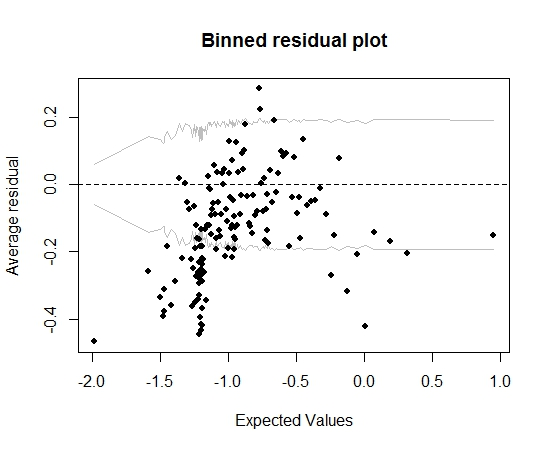# Assessing logistic regression models

This question arises from my actual confusion about how to decide if a logistic model is good enough. I have models that use the state of pairs individual-project two years after they are formed as a dependent variable. The outcome is successful (1) or not (0). I have independent variables measured at the time of formation of the pairs. My aim is to test whether a variable, which I hypothesized would influence the success of the pairs has an effect on that success, controlling for other potential influences. In the models, the variable of interest is significant.

The models were estimated using the glm() function in R. To assess the quality of the models, I have done a few things: glm() gives you the residual deviance, the AIC and the BIC by default. In addition, I have calculated the error rate of the model and plotted the binned residuals.

• The complete model has a smaller residual deviance, AIC and BIC than the other models that I have estimated (and that are nested in the complete model), which leads me to think that this model is "better" than the others.
• The error-rate of the model is fairly low, IMHO (as in Gelman and Hill, 2007, pp.99):
error.rate <- mean((predicted>0.5 & y==0) | (predicted<0.5 & y==1), at around 20%.

So far so good. But when I plot the binned residual (again following Gelman and Hill's advice), a large portion of the bins fall outside of the 95% CI:That plot leads me to think there is something utterly wrong about the model. Should that lead me to throw the model away? Should I acknowledge that the model is imperfect but keep it and interpret the effect of the variable of interest? I have toyed around with excluding variables in turn, and also some transformation, without really improving the binned residuals plot.

Edit:

• At the moment, the model has a dozen predictors and 5 interaction effects.
• The pairs are "relatively" independent of each other in the sense that they are all formed during a short period of time (but not stricly speaking, all simultaneously) and there are a lot of projects (13k) and a lot of individuals (19k), so a fair proportion of projects are only joined by one individual (there are about 20000 pairs).
• A rough estimate of the minimum sample size needed to do what you are doing is that you need the minimum of the number of events or the number of non-events to exceed 15 times (12 + 5), assuming you had 17 candidate terms in the model. If you did any screening of predictors, making use of $Y$, all bets are off. – Frank Harrell Nov 12 '11 at 13:35
• Based on what you say, the sample size doesn't seem to be a problem, because I have about 20000 thousand pairs (of which roughly 20% are successful). – Antoine Vernet Nov 12 '11 at 14:57

Classification accuracy (error rate) is an improper scoring rule (optimized by a bogus model), arbitrary, discontinuous, and easy to manipulate. It's not needed in this context.

You didn't state how many predictors there were. Instead of assessing model fit I would be tempted to just make the model fit. A compromise approach is to assume that interactions aren't important and to allow continuous predictors to be nonlinear using regression splines. Plot the estimated relationships. The rms package in R makes all this relatively easy. See http://biostat.mc.vanderbilt.edu/rms for more information.

You might elaborate on "pairs" and whether your observations are independent.

• If I understand correctly, your advice is to concentrate on the plot of the residuals and get this one straight before doing anything else, eventually using regression splines, am I correct? I edited the question to state how many predictors there are and that the pairs are "relatively" independent. – Antoine Vernet Nov 12 '11 at 13:04
• Hi @Frank. Why do you say classification accuracy is a bad method? Is that because it is, here, assessed on the same data where the model is derived? – Peter Flom Nov 12 '11 at 13:11
• No my comment was not to concentrate on residual plots but rather to make the model fit "up front". Peter the proportion classified "correctly" has multiple problems, including the fact that it is the lowest precision estimator of predictive performance, is arbitrary, and will lead to selection of the wrong model. I have an example where adding a very strong predictor (on the basis of likelihood ratio $\chi^2$ or $c$-index (ROC area) makes the proportion classified correctly decrease significantly. – Frank Harrell Nov 12 '11 at 13:37
• No, I mean allowing continuous predictors to operate nonlinearly on the log odds scale, by expanding them into multiple terms using restricted cubic splines (natural splines). Then plot the estimated transformations to learn about partial effects of each predictor. – Frank Harrell Nov 13 '11 at 14:08
• Thanks for the input, that was really helpful. Going more in depth with the data, I realized that I had collinearity issues (even if I had no high pairwise correlations). – Antoine Vernet Jan 18 '12 at 10:28

The situation does seem a bit strange, but I think your plot may provide a clue. It looks like there may be a curvilinear relationship. It is permissible to use polynomial terms and other transformations of predictor variables (e.g., $x^2$) in logistic regression, just as in OLS regression. This might be worth a try.